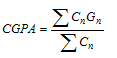# GRADING AND POINT SYSTEM

GRADING AND POINT SYSTEM (As directed by UGC):

 Numerical Grade Letter Grade Grade Point 80% -100% A+ (A Plus) 4.0 75% – 79.99% A (A Regular) 3.75 70% – 74.99% A- ( A Minus) 3.5 65% – 69.99% B+ (B Plus) 3.25 60% – 64.99% B (B Regular) 3.0 55% – 59.99% B- ( B Minus) 2.75 50% -54.99% C+ (C Plus) 2.5 45% – 49.99% C (C Regular) 2.25 40% – 44.99% D – 2.0 Less than 40% F – 0.0

Note: If Letter Grade ‘I’ (Incomplete) is awarded to any student in any course, it indicates that he/she has attended the course but did not complete all requirements of the course. Letter Grade “W” indicates withdrawn from the course.

CUMULATIVE GRADE POINT AVERAGE

Two kinds of grade point average are used at IBAIS University. The CGPA is calculated by dividing the total earned grade points by the number of credit hours attempted in a given trimester. The earned grade point, is computed by multiplying the value of the grade point equivalent to the corresponding letter grade by the number of credit hours for the course (e.g. a student earning a B (3.0) grade in a 3 credit hours course earns 9.0 quality points).

The Cumulative GPA is calculated by the following equation:Where, C is Credit Hour, G is Grade Point. So,and

It is necessary to maintain a certain CGPA for various degrees at the University. For example, a CGPA of C+ (2.5) for Bachelor degree and B (3.0) for Master’s degree is essential.